# Finding Benchmark Fractions and Percentages for a Figure Online Quiz

Following quiz provides Multiple Choice Questions (MCQs) related to Finding Benchmark Fractions and Percentages for a Figure. You will have to read all the given answers and click over the correct answer. If you are not sure about the answer then you can check the answer using Show Answer button. You can use Next Quiz button to check new set of questions in the quiz.Q 1 - What percent of the grid is shaded? Choose the closest benchmark percent.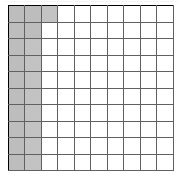### Explanation

Step 1:

Given is a 10 × 10 grid having 100 squares. The number of shaded squares gives the percentage of the grid.

Step 2:

So, the percent of the grid that is shaded is 21%.

Step 3:

For 21%, the closest benchmark percent is 20%

Q 2 - What percent of the grid is shaded? Choose the closest benchmark percent.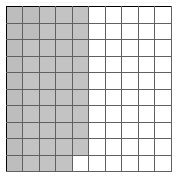### Explanation

Step 1:

Given is a 10 × 10 grid having 100 squares. The number of shaded squares gives the percentage of the grid.

Step 2:

So, the percent of the grid that is shaded is 49%.

Step 3:

For 49%, the closest benchmark percent is 50%

Q 3 - What percent of the grid is shaded? Choose the closest benchmark percent.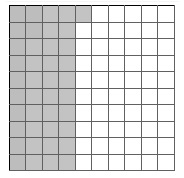### Explanation

Step 1:

Given is a 10 × 10 grid having 100 squares. The number of shaded squares gives the percentage of the grid.

Step 2:

So, the percent of the grid that is shaded is 41%.

Step 3:

For 41%, the closest benchmark percent is 40%

Q 4 - What percent of the grid is shaded? Choose the closest benchmark percent.### Explanation

Step 1:

Given is a 10 × 10 grid having 100 squares. The number of shaded squares gives the percentage of the grid.

Step 2:

So, the percent of the grid that is shaded is 21%.

Step 3:

For 66%, the closest benchmark percent is $66\frac{2}{3}\%$

Q 5 - What percent of the grid is shaded? Choose the closest benchmark percent.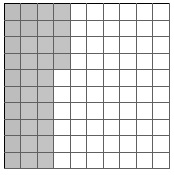### Explanation

Step 1:

Given is a 10 × 10 grid having 100 squares. The number of shaded squares gives the percentage of the grid.

Step 2:

So, the percent of the grid that is shaded is 34%.

Step 3:

For 34%, the closest benchmark percent is $33\frac{1}{3}\%$

Q 6 - What percent of the grid is shaded? Choose the closest benchmark percent.### Explanation

Step 1:

Given is a 10 × 10 grid having 100 squares. The number of shaded squares gives the percentage of the grid.

Step 2:

So, the percent of the grid that is shaded is 74%.

Step 3:

For 74%, the closest benchmark percent is 75%

Q 7 - What percent of the grid is shaded? Choose the closest benchmark percent.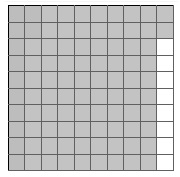### Explanation

Step 1:

Given is a 10 × 10 grid having 100 squares. The number of shaded squares gives the percentage of the grid.

Step 2:

So, the percent of the grid that is shaded is 92%.

Step 3:

For 92%, the closest benchmark percent is 90%

Q 8 - What percent of the grid is shaded? Choose the closest benchmark percent.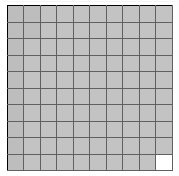### Explanation

Step 1:

Given is a 10 × 10 grid having 100 squares. The number of shaded squares gives the percentage of the grid.

Step 2:

So, the percent of the grid that is shaded is 99%.

Step 3:

For 99%, the closest benchmark percent is 100%

Q 9 - What percent of the grid is shaded? Choose the closest benchmark percent.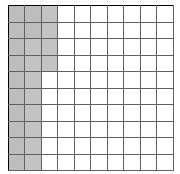### Explanation

Step 1:

Given is a 10 × 10 grid having 100 squares. The number of shaded squares gives the percentage of the grid.

Step 2:

So, the percent of the grid that is shaded is 24%.

Step 3:

For 24%, the closest benchmark percent is 25%

Q 10 - What percent of the grid is shaded? Choose the closest benchmark percent.### Explanation

Step 1:

Given is a 10 × 10 grid having 100 squares. The number of shaded squares gives the percentage of the grid.

Step 2: